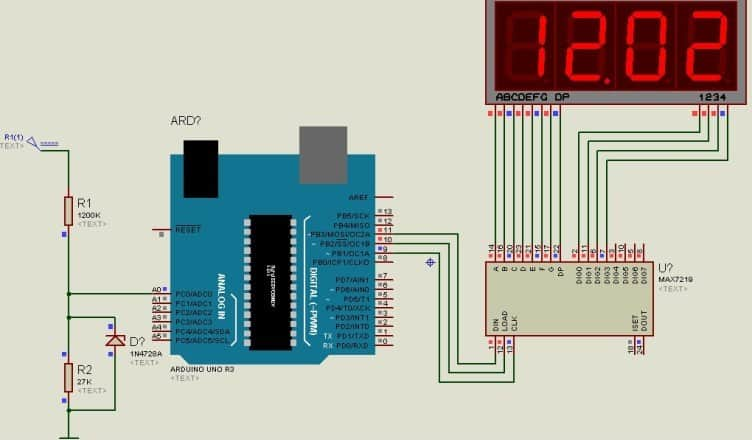0-50V DC Voltmeter using Arduino and Seven Segement Display(Last Updated On: February 17, 2019)

Introduction:

We are back again here with the latest Arduino Project, i.e DC Voltmeter using Arduino and Seven Segment Display. This time we will be interfacing Arduino with 7 segment display and MA7219 IC to measure voltage ranging from 0-50V with almost no error at all. This DC voltmeter is linear with voltage and shows a linear characteristics from 0.01 Volt to 50 Volt. Voltage beyond 50 Volt isn’t measurable by it. The above figure shows the 0-50V DC Voltmeter using Arduino and Seven Segment Display.

The Arduino inbuilt 10 bit ADC, can be used for measuring the 0-50V. Popular MAX7219 display driver, connected with Arduino Uno is used for displaying the measured DC voltage.

Parts & Components:

```1. Arduino UNO Board
2. MA7219 IC
3. Resistors – 27K & 1200K
4. 1N4728 3.3 Volt Zener Diode
5. 4 digit, common cathode Seven Segment Display```

Design & Explanation:

The voltage divider circuit is used to divide voltage into two parts, out of which one is fed to the input analog terminal of Arduino. Internal reference voltage as Vref 1.1V of Arduino is selected for measurement.

The resistor R1and R2 forms the voltage divider which are connected to analog input pin A0 of Arduino.
1N4728 3.3 Volt Zener Diode, at A0 pin is used for high voltage protection for Arduino.

Vout = (R2 / R1+R2 ) * Vin.

If Vin is 50V then

Vout = (27/1200+27) * 50V = 1.1 V = 1100 mV.
ADC Resolution = Vref / (1024 -1)
ADC Resolution = 1.1 V / 1023 = 1100mV / 1023 = 1.075 mV

0-50V DC Voltmeter using Arduino and Seven Segement Display:0-50V DC Voltmeter using Atemga328 and Seven Segement Display:

The same project can also be implemented using Atmega328 micro-controller. All you need to do is replace the Arduino Board with Atmega328 micro-controller, with addition of few more components as in the circuit diagram below.Programming & Source Code:

The programming is written in Arduino language and same program is applicable for both circuits. For first circuit you can directly upload the program. And for 2nd circuit you need to upload program to ATmega328. This is done by replacing ATmega328 micro-controller on Arduino Uno Board with fresh ATmega328 micro-controller. Before uploading you need to upload bootloader, then only program can be successfully uploaded.

```#include <LedControl.h>

int analogPin1 = 0;
int val = 0;

// inputs: DIN pin, CLK pin, LOAD pin. number of chips
LedControl mydisplay = LedControl(11, 9, 10, 1);

void setup()
{
analogReference(INTERNAL);
mydisplay.shutdown(0, false);  // turns on display
mydisplay.setIntensity(0, 15); // 15 = brightest
mydisplay.setDigit(0, 0, 9, false);
mydisplay.setDigit(0, 1, 8, false);
mydisplay.setDigit(0, 2, 7, false);
mydisplay.setDigit(0, 3, 6, false);
mydisplay.setDigit(0, 4, 5, true);
mydisplay.setDigit(0, 5, 4, false);
mydisplay.setDigit(0, 6, 3, false);
mydisplay.setDigit(0, 7, 2, false);
}

void loop()
{
int converted = map(val, 0, 1023, 0,5000);

converted = HexToBCD(converted);

mydisplay.setDigit(0, 0, ((converted>>12)&0x0F), false);
mydisplay.setDigit(0, 1, ((converted>>8)&0x0F), true);
mydisplay.setDigit(0, 2, ((converted>>4)&0x0F), false);
mydisplay.setDigit(0, 3, (converted&0x0F), false);

delay(1000);
}
unsigned int HexToBCD(unsigned int number)
{
unsigned char i=0;
unsigned int k = 0;

while(number)
{
k = ( k ) | ((number%10) << i*4);
number = number / 10;
i++;
}

return(k);
}```

Recommended For You